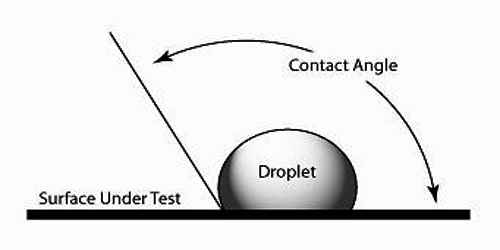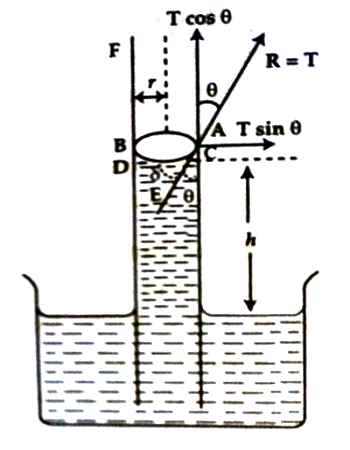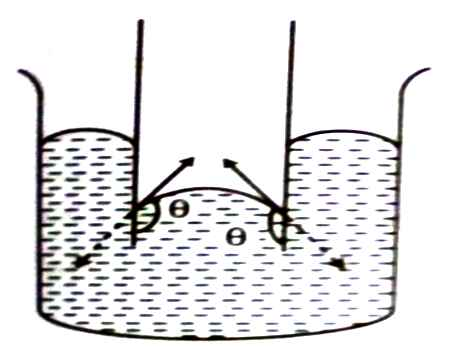Physics

# Explanation: Water with Capillary Tube Depending on Angle of ContactEvent of ascending of water in a capillary tube depending on Angle of Contact:

Capillary tube is a tube having uniform fine bore. When it is dipped partially inside water, then water rises in the capillary tube. The reason for this rise is discussed below:

It has been seen from experiment that when a capillary tube is dipped partially inside water, then water rises slightly. Again, when it is dipped in mercury, then level of mercury drops to a little amount. The reason for these phenomena is:From figure, the vertical component of the reaction force, T between glass and water = T cos θ. Since contact angle θ being acute angle (0 < θ < 90°), so, T cos θ is positive. Besides, horizontal component T sin θ being active on the two ends of the tube in opposite direction, so cancels out action of each other. So, due to vertical force T cos θ, water rises through the capillary tube. Principal theory for the determination of surface tension of water by capillary tube method,

T = [rρg (h + ½)] / [2 cos θ] … … … (1)

Here, r = radius of the tube, ρ = density of water, h = height of water level inside the tube, θ = angle of contact.In figure- 2, the capillary tube has been dipped inside mercury. In this case angle of contact is obtuse angle (90° < 0 < 180°). From the direction of surface tension and reaction force, it is seen that there is no vertical component of the reaction force. One downward component exists. Due to this downward force, level of mercury goes down. This phenomenon can also be explained in the following way:

Since θ is obtuse angle, so cos θ is negative. Now from the equation (1) of surface tension it is seen that if cos θ is negative, then right hand side of the equation will be negative, but T of left hand side is positive. So, if cos θ is negative, then it becomes negative. This means that mercury level goes down inside the glass tube.